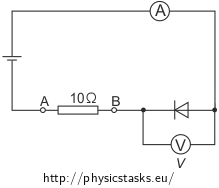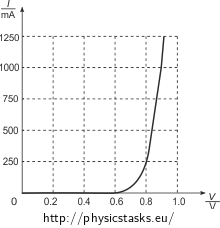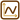## Semiconductor Diode in Forward Direction

### Task number: 1777

A semiconductor silicone diode is connected in the forward direction in an electric circuit according to the Figure on the left.

1. What is the reading on the voltmeter if the ammeter indicates 250 mA?
2. Asses the voltage between points A and B (i.e. the voltage across the resistor) and the terminal voltage of the battery.
3. What voltage across the diode will cause a current of 1000 mA through the battery?

The plot on the right shows the I-V characteristics of the diode in the forward direction.We presume in this task that both the devices are ideal, i.e. the voltmeter has an infinite resistance and the ammeter has a zero resistance.

• #### Solution of Part a.

The current through the diode is not directly proportional to the voltage across the diode, Ohm’s law does not hold. We have to read the voltage across the diode for a given current from the I-V plot. Since the voltmeter is an ideal one, the same current flows through the whole circuit. The ammeter thus indeed indicates the magnitude of the current through the diode, the baterry and the resistor.

Invalid enlarge filename!

From the current-voltage characteristics we see that a current of ID = 250 mA through the diode corresponds to a voltage of UD = 0.8 V across the diode.

• #### Solution of Part b.Resistance of the resistor R:

$R\,=\,10\,\mathrm{\Omega}\,.$

The same current is flowing through the ammeter, the diode and the resistor.

$I\,=\,250\,\mathrm{mA}\,=\,0.25\,\mathrm{A}\,.$

We calculate the voltage across the resistor from the Ohm’s law:

$U_R\,=\,RI\,.$

where R is the resistance of the resistor and I is the current flowing through the resistor.

$U_R\,=\,10 \,\cdot\, 0.25\,\mathrm{V}\,=\,2.5\,\mathrm{V}\,.$

The terminal voltage of the baterry is equal to the sum of the voltages across the resistor and across the diode:

$U\,=\,U_R+U_D$ $U\,=\,\left(2.5+0.8\right)\,\mathrm{V}\,=\,3.3\,\mathrm{V}\,.$
• #### Solution of Part c.

The current flowing through the baterry is the same as the current flowing through the diode. Therefore we read the voltage across the diode from the plot:

The current of I = 1000 mA through the baterry corresponds to a voltage across the diode of UD = 0.9 V.